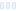# Weight Loss Formula, Chocolate

## Diet Doc

Main info:

Weight Loss Formula, Chocolate
Diet Doc
1 scoop
85 Calories
7 g
2.5 g
9.5 g

3.5 g
0 mg
0 g
65 mg
1 g
0 g

Nutrition Facts
For a Serving Size of (g)
How many calories are in Weight Loss Formula, Chocolate? Amount of calories in Weight Loss Formula, Chocolate: Calories Calories from Fat (%)
% Daily Value *
How much fat is in Weight Loss Formula, Chocolate? Amount of fat in Weight Loss Formula, Chocolate: Total Fat
How much sodium is in Weight Loss Formula, Chocolate? Amount of sodium in Weight Loss Formula, Chocolate: Sodium
How many carbs are in Weight Loss Formula, Chocolate? Amount of carbs in Weight Loss Formula, Chocolate: Carbohydrates
How many net carbs are in Weight Loss Formula, Chocolate? Amount of net carbs in Weight Loss Formula, Chocolate: Net carbs
How much sugar is in Weight Loss Formula, Chocolate? Amount of sugar in Weight Loss Formula, Chocolate: Sugar
How much fiber is in Weight Loss Formula, Chocolate? Amount of fiber in Weight Loss Formula, Chocolate: Fiber
How much protein is in Weight Loss Formula, Chocolate? Amount of protein in Weight Loss Formula, Chocolate: Protein
Vitamins and minerals
How much Vitamin A is in Weight Loss Formula, Chocolate? Amount of Vitamin A in Weight Loss Formula, Chocolate: Vitamin A
How much Vitamin C is in Weight Loss Formula, Chocolate? Amount of Vitamin C in Weight Loss Formula, Chocolate: Vitamin C
How much Calcium is in Weight Loss Formula, Chocolate? Amount of Calcium in Weight Loss Formula, Chocolate: Calcium
How much Iron is in Weight Loss Formula, Chocolate? Amount of Iron in Weight Loss Formula, Chocolate: Iron
Fatty acids
Amino acids
* The Percent Daily Values are based on a 2,000 calorie diet, so your values may change depending on your calorie needs.
Percent calories from...Loading similar foods...
Note: Any items purchased after clicking our Amazon buttons will give us a little referral bonus. If you do click them, thank you!Be cool

- Zen orangeI never skip arm day

- Buff broccoli